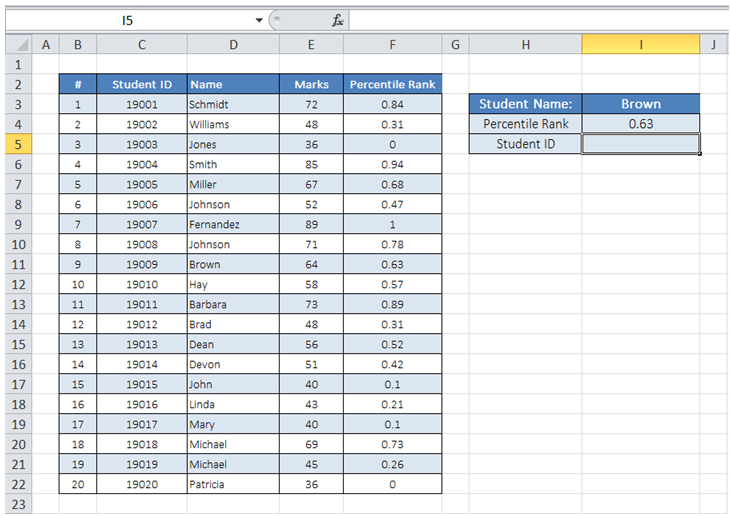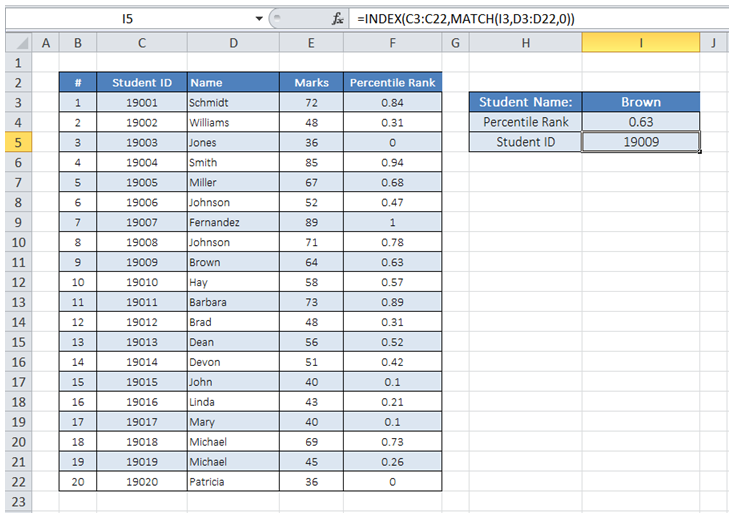# Excel Made Easy

## Vlookup retrieve value to the right in Excel

For example, I want to enter a Student Name in cell I3 and get the corresponding Percentile Rank information pulled from the table in cell I4 and Student ID information in cell I5..

I can use VLOOKUP for retrieving Percentile Rank value. However, since Student ID column is to the left of Name Column, I am not able to use VLOOKUP.To do it in Excel, here is the answer:

a) Enter the formula =INDEX(C3:C22,MATCH(I3,D3:D22,0)) in cell I5 where ID for the student selected is required.

The first argument of MATCH "I3" has the Lookup value - the Student name whose ID is to be pulled from the Table.

The second argument of MATCH "D3:D22" is the array range containing the lookup value.

The 3rd argument "0' sets up the function to seek an exact match to the value entered in cell I3.

The Match function returns an Index number of the lookup value in the array.

The Index function then takes this index as its second argument and returns a value from the array specified in its first argument(C3:C22).